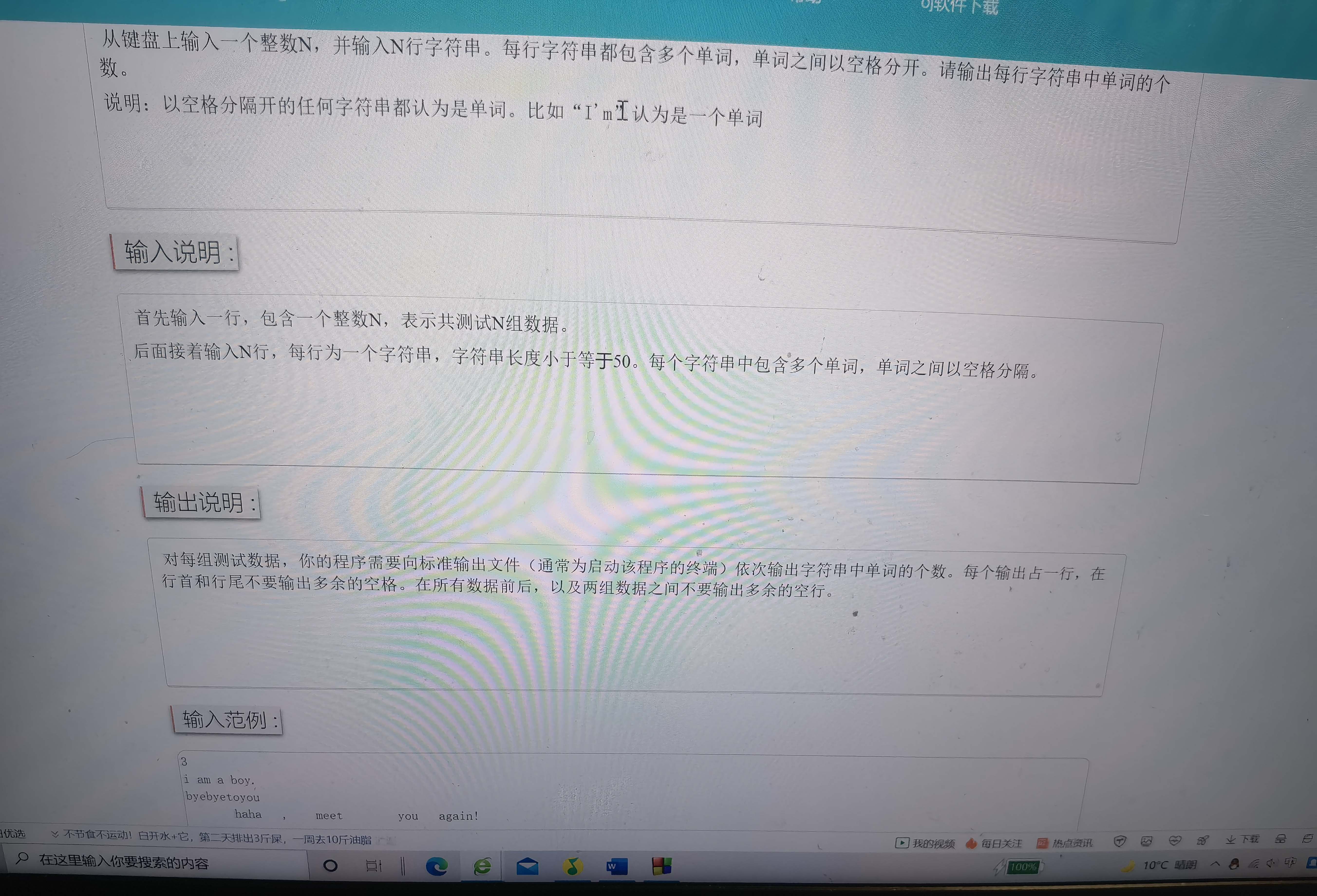2021-11-25 23:49

# 一提标准的大一oj提木• 好问题 提建议
• 收藏

#### 2条回答默认 最新

•ByteSniper 2021-11-26 00:47
已采纳
``````#include <iostream>
#include <string>
using namespace std;
int countSegments(string s)
{
s.push_back(' ');
int cnt = 0;
for (unsigned int i = 0; i < s.size() - 1; i++)
{
if (s[i] != ' ' && s[i + 1] == ' ') cnt++;
}
return cnt;
}
int main()
{
int N = 0;
string s = "";
cin >> N;
getchar();
for (int i = 0; i < N; i++)
{
getline(cin, s);
cout << countSegments(s) << endl;
}
return 0;
}
``````
已采纳该答案
评论
解决 无用
打赏 举报
•校歪歪 2021-11-26 10:30
``````#include <iostream>
#include <string>
using namespace std;
size_t word_count(string s)
{
size_t count = 0;
for (size_t i=0;i<s.length();i++)
{
if((s[i]>=65&&s[i]<=90)||(s[i]>=97&&s[i]<=122))
{
count++;
while(((s[i]>=65&&s[i]<=90)||(s[i]>=97&&s[i]<=122))&&s[i]!='\0')
{
i++;
}
}
}
return count;
}
int main()
{
int N = 0;
string s = "";
cin >> N;
getchar();
for (int i = 0; i < N; i++)
{
getline(cin, s);
cout<<word_count(s)<<endl;
}
return 0;
}

``````

编辑记录

评论
解决 无用
打赏 举报